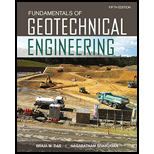Chapter 13, Problem 13.20PFundamentals of Geotechnical Engin...

5th Edition
Braja M. Das + 1 other
ISBN: 9781305635180

Solutions

Chapter
SectionFundamentals of Geotechnical Engin...

5th Edition
Braja M. Das + 1 other
ISBN: 9781305635180
Textbook Problem

Refer to Figure 13.38. Use Taylor’s chart for ϕ ′ > 0 (Figure 13.17) to find the critical height of the slope in each case:a. n′ = 2, ϕ ′ = 15 ° , c′ = 31.1 kN/m2, and γ = 18.0 kN/m3b. n′ = 1, ϕ ′ = 25 ° , c′ = 24 kN/m2, and γ = 18.0 kN/m3c. n′ = 2.5, ϕ ′ = 12 ° , c′ = 25 kN/m2, and γ = 17 kN/m3d. n′ = 1.5, ϕ ′ = 18 ° , c′ = 18 kN/m2, and γ = 16.5 kN/m3FIG. 13.38

(a)

To determine

Find the critical height of the slope.

Explanation

Given information:

The bed slope (n) is 2.

The angle (ϕ) of friction is 15°.

The cohesion (c) is 31.1kN/m2.

The unit weight of the soil (γ) is 18.0kN/m3.

Calculation:

Calculate slope angle (β) using the equation:

β=tan1(1n)

Substitute 2 for n.

β=tan1(12)=26.57°

Find the Taylor’s stability number (m).

Refer Figure 13.17, “Taylor’s stability number” in the textbook

(b)

To determine

Find the critical height of the slope.

(c)

To determine

Find the critical height of the slope.

(d)

To determine

Find the critical height of the slope.

Still sussing out bartleby?

Check out a sample textbook solution.

See a sample solution

The Solution to Your Study Problems

Bartleby provides explanations to thousands of textbook problems written by our experts, many with advanced degrees!

Get Started

Find more solutions based on key concepts